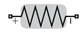# Resistor

Model resistor for circuit envelope analysis

• Library:
• RF Blockset / Circuit Envelope / Elements

•## Description

The Resistor block models a resistor within the RF Blockset™ circuit envelope simulation environment. For an introduction to RF simulation, see the example, Simulate High Frequency Components.

The block implements the relation

`$v\left(t\right)=Ri\left(t\right)$`

where:

• R represents the resistance, as a function of temperature.

• i(t) represents the current through the capacitor at time t.

• v(t) represents the voltage across the terminals of the capacitor at time t.

RF Blockset current and voltage signals consist of in-phase (Ik) and quadrature (Qk) components at each frequency fk specified in the Configuration block:

`$\begin{array}{c}i\left(t\right)=\sum _{\left\{{f}_{k}\right\}}\left({i}_{{I}_{k}}\left(t\right)+j\cdot {i}_{{Q}_{k}}\left(t\right)\right){e}^{j\left(2\pi {f}_{k}\right)t}\\ v\left(t\right)=\sum _{\left\{{f}_{k}\right\}}\left({v}_{{I}_{k}}\left(t\right)+j\cdot {v}_{{Q}_{k}}\left(t\right)\right){e}^{j\left(2\pi {f}_{k}\right)t}\end{array}$`

## Parameters

expand all

Resistance value , specified as a real number in ohms greater than zero. Specify the units of the resistance from the corresponding drop-down menu.

Select this parameter, to simulate thermal noise in a resistor. Then, in the Configuration block dialog box, also select the Simulate noise check box. By default, both Simulate noise check boxes are selected.

This parameter inserts a current noise source with the single-sided power density of 4 kT/R A2/Hz, where:

• k is the Boltzmann constant

• T is the value of the Temperature parameter, in degrees Kelvin. (Also located in the Configuration block.)